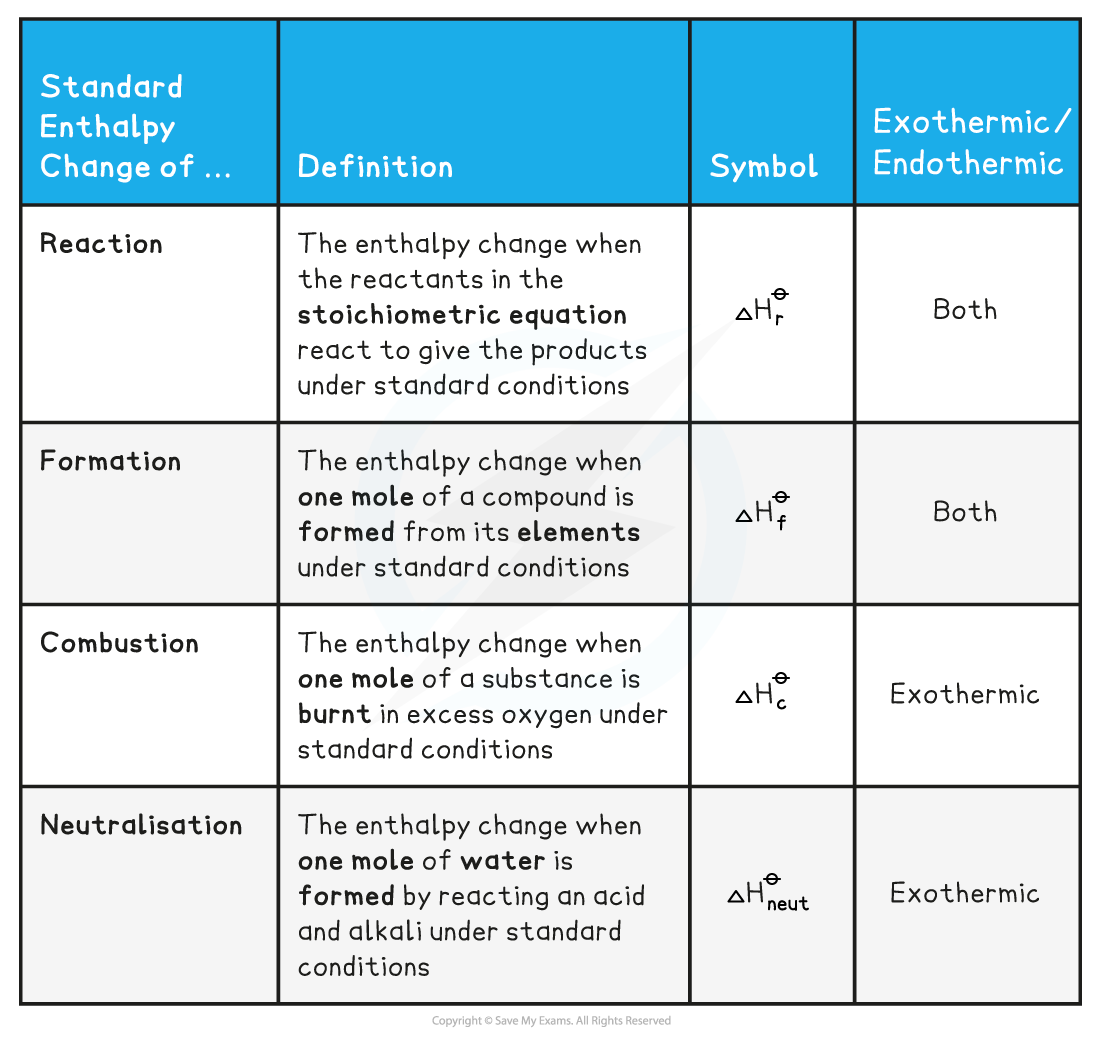# IB DP Chemistry: SL复习笔记5.1.2 Standard Enthalpy Change

### Standard Enthalpy Change

#### Standard conditions

• To compare the changes in enthalpy between reactions, all thermodynamic measurements are made under standard conditions
• These standard conditions are:
• pressure of 100 kPa
• concentration of 1 mol dm-3 for all solutions
• Each substance involved in the reaction is in its standard state (solid, gas or liquid)
• Temperature is not part of the definition of standard state, but a temperature of 298 K (25 oC) is usually given as the specified temperature
• To show that a reaction has been carried out under standard conditions, the symbol  is used
• Eg. ΔH = the standard enthalpy change

#### Standard Enthalpies

• There are a few Standard Enthalpy changes which are used commonly in energy calculations and they are summarised below:• Practice your understanding of enthalpy changes on the following worked examples:

#### Worked Example

One mole of water is formed from hydrogen and oxygen releasing 286 kJ

H2 (g) + ½O2 (g)  → H2O (l)             ΔHr= -286 kJ mol-1

Calculate ΔHr for the reaction below:2H2 (g) + O2 (g)   H2O (l)

• Since two moles of water molecules are formed in the question above, the energy released is simply:

ΔHr = 2 mol x (-286 kJ mol-1)= - 572 kJ

#### Worked Example

Calculate ΔHr for the reaction below

4Fe (s) +O2 (g)    2Fe2O3 (s)

given that ΔHꝊ [Fe2O3 (s)]  = - 824 kJ mol-1

• Since two moles of Fe2O3 (s) are formed the total change in enthalpy for the reaction above is:

ΔHf =  2 mol x ( -824 kJ mol-1)= - 1648 kJ

#### Worked Example

Identify each of the following as  ΔHr, ΔHf, ΔHc or ΔHneut

1. MgCO(s)  MgO (s) + CO2 (g)
2. C (graphite) + O2 (g)  CO2 (g)
3. HCl (aq) + NaOH (aq)   NaCl (aq) + H2O (l)

Answer 2: ΔHf as one mole of CO2 is formed from its elements in standard state and ΔHc as one mole of carbon is burnt in oxygen

Answer 3: ΔHneut as one mole of water is formed from the reaction of an acid and alkali

#### Exam Tip

You need to learn well the Standard Enthalpy change definitions as they are frequently tested in exam papers